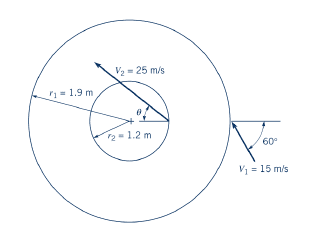# Turbomachinery velocity triangle problem

Gold Member## Homework Statement

Air (assumed incompressible) flows across the rotor shown in the figure such that the magnitude of the absolute velocity increases from 15 m/s to 25 m/s. Measurements indicate that the absolute velocity at the inlet is in the direction shown. Determine the direction of the absolute velocity at the outlet if the fluid puts no torque on the rotor. Is the rotation CW or CCW? Is this device a pump or a turbine?

## Homework Equations

Euler's Torque equation

$T=\dot{m}(r_2V_{\theta2}-r_1V_{\theta1})$

## The Attempt at a Solution

[/B]
As torque = 0

$T=\dot{m}(r_2V_{\theta2}-r_1V_{\theta1})=0$

$(r_2V_{\theta2})=(r_1V_{\theta1})$

$V_{\theta2}=V_{\theta1}*\frac{r_1}{r_2}=15\sin{60}*\frac{1.9}{1.2}=20.57 m/s$

No idea where to take it from here. I can't tell from the problem statement if the direction of $V_2$ is as indicated.

If there is no torque, as indicated, then I would assume that this must be a pump--as the fluid isn't doing any work on the rotor. However, I believe I need to prove this with velocity triangles and the like.

Any help would be appreciated.
Mac